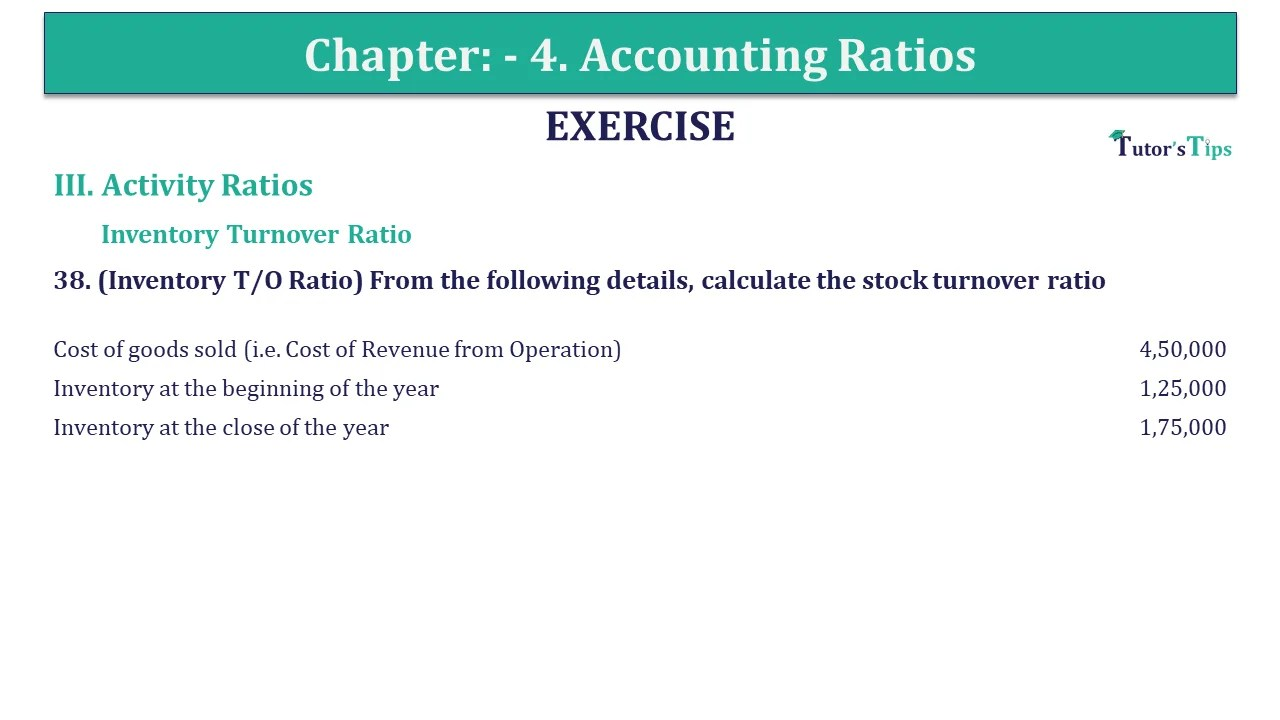# Question 38 Chapter 4 of +2-B – USHA Publication 12 ClassQ-38- CH-4 Book 2 - Usha Pub. +2 Book 2020 - Solution

Question 38 Chapter 4 of +2-B

III. Activity Ratios

Inventory Turnover Ratio

38. (Inventory T/O Ratio) From the following details, calculate the stock turnover ratio

 Cost of goods sold (i.e. Cost of Revenue from Operation) 4,50,000 Inventory at the beginning of the year 1,25,000 Inventory at the close of the year 1,75,000

## The solution of Question 38 Chapter 4 of +2-B: –

 Inventory Turnover Ratio = Cost of goods sold Average Inventory
 Cost of goods sold = Net Total Sales – Gross profit = ₹ 4,50,000
 Debt Equity Ratio = Opening Inventory – Closing Inventory 2 = ₹ 1,25,000 + ₹ 1,75,000 2 = ₹ 1,50,000

 Inventory Turnover Ratio = ₹ 4,50,000 ₹ 1,50,000 = 3 Times

Also, Check out the solved question of previous Chapters: –